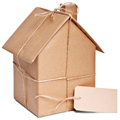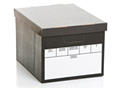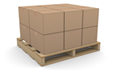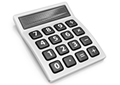This guide to estimating storage size gives you a rough idea of how much room you’re likely to need. Our friendly staff will be glad to help you calculate the exact size of storage unit you need.

### Houses / Flat

 1 bedroom = 30-50 sq ft unit2 bedroom = 50-80 sq ft unit 3 bedroom = 100-150 sq ft unit 4 bedroom = 150-250 sq ft unit

### Vehicles

 Transit Van = 35-50 sq ft unitLuton Van = 70-80 sq ft unit 7 1/2 ton Van = 140-170 sq ft unit 20ft container = 170-250 sq ft unit

### Archive boxes

50 boxes =  25 sq ft unit100 boxes =  50 sq ft unit
150 boxes =  75 sq ft unit
200 boxes =  100 sq ft unit
300 boxes =  150 sq ft unit

### Pallets

1 pallet (unloaded) =  16 sq ft unit2 pallets (unloaded) =  25 sq ft unit
6 pallets (loaded) =  125 sq ft unit
7 pallets (loaded) =  150 sq ft unit
9 pallets (loaded) =  200 sq ft unit
11 pallets (loaded) =  250 sq ft unit

### Conversion Calculator

25 sq ft =  2.3 sqm50 sq ft =  4.6 sqm
75 sq ft =  7.0 sqm
100 sq ft =  9.3 sqm
150 sq ft =  13.9 sqm
200 sq ft =  18.6 sqm